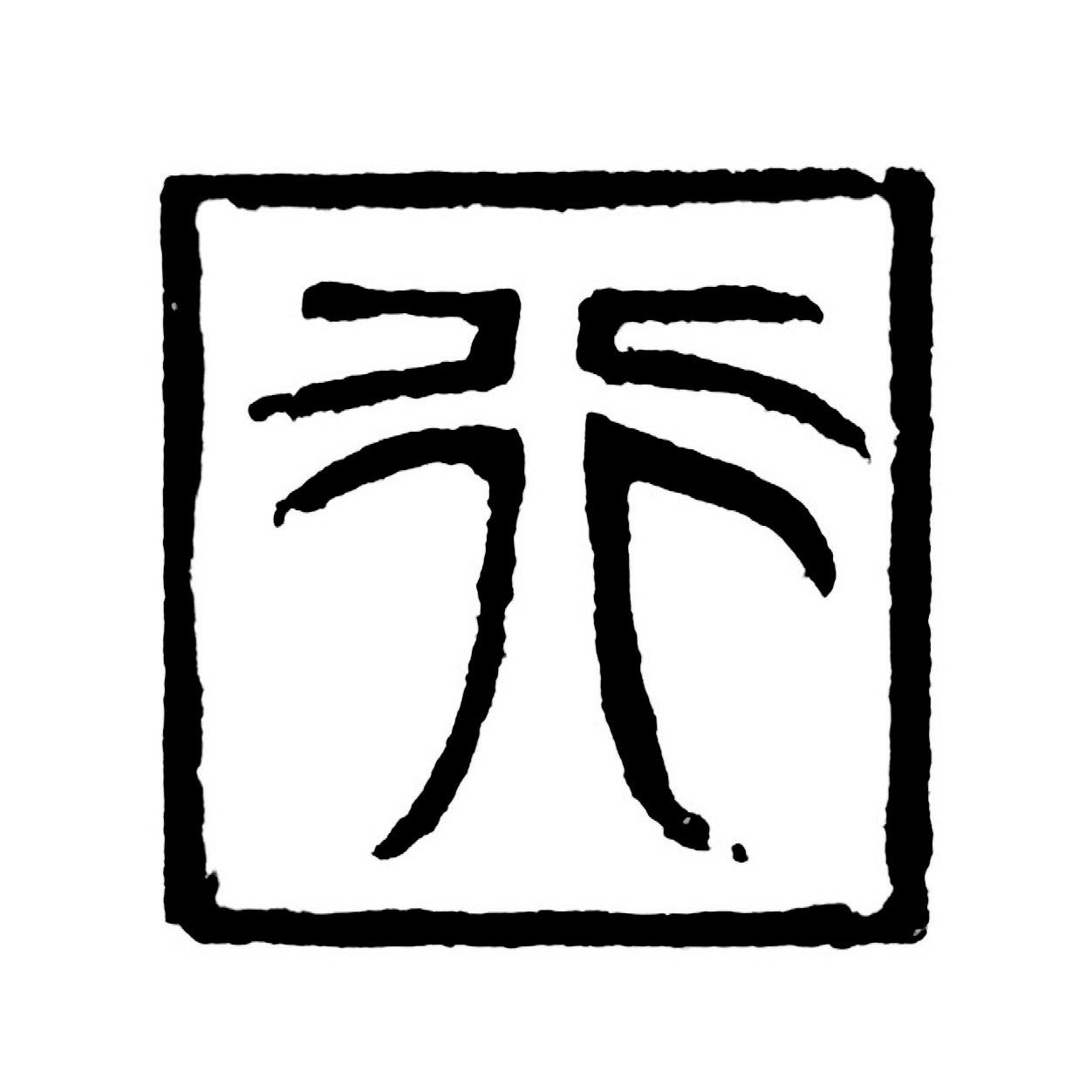二十二画程序员293 0 0

# 0. 前言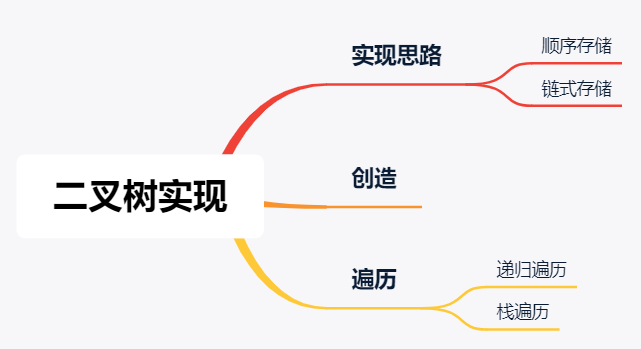# 1. 二叉树的实现思路

## 1.0. 顺序存储——数组实现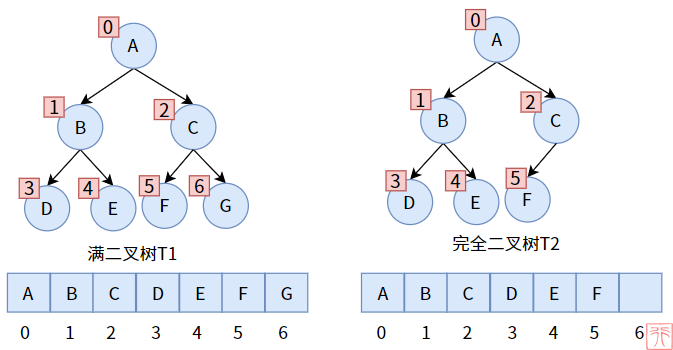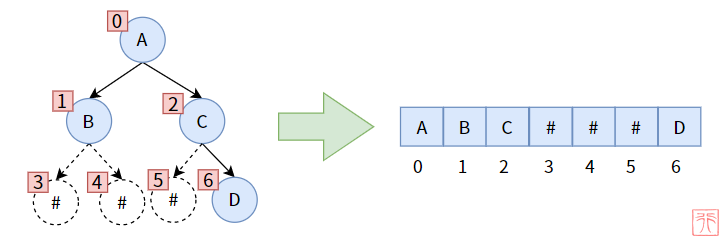## 1.1. 链式存储——链表实现

• 数据元素
• 左子树分支（结点的左孩子）
• 右子树分支（结点的右孩子）

• 一个存储数据的变量——data
• 一个指向其左孩子结点的指针——left_child
• 一个指向其右孩子结点的指针——right_child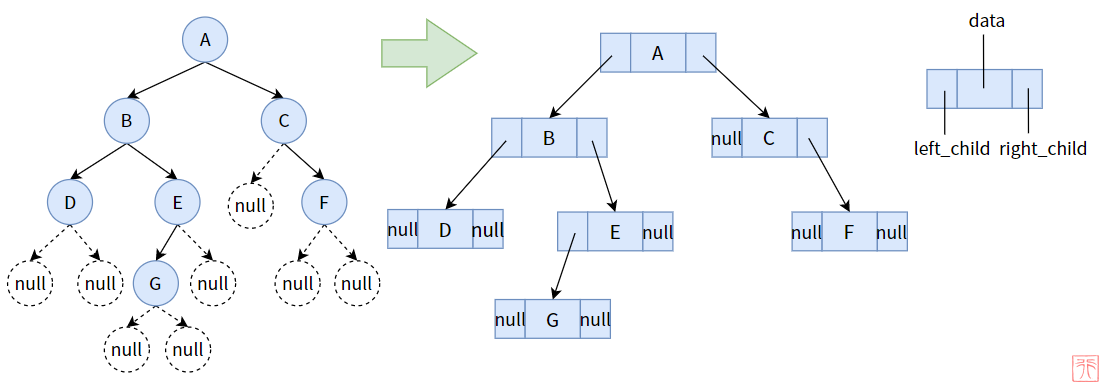/*二叉树的结点的结构体*/
typedef struct Node {
char data; //数据域
struct Node *left_child; //左孩子指针
struct Node *right_child; //右孩子指针
} TreeNode;

# 2. 二叉树的创造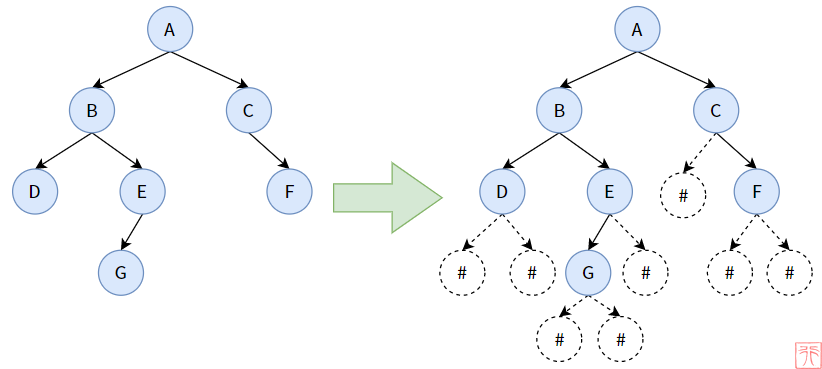/**
* 创造一个二叉树
* root: 指向根结点的指针的指针
*/
void create_binary_tree(TreeNode **root)
{
char elem;
scanf("%c", &elem);
if (elem == '#') {
*root = NULL;
} else {
*root = create_tree_node(elem); //创造一个二叉结点
create_binary_tree(&((*root)->left_child));
create_binary_tree(&((*root)->right_child));
}
}

# 3. 二叉树的遍历

## 3.1. 递归实现

【先序遍历】

/**
* 先序遍历
* root: 指向根结点的指针
*/
void preorder_traversal(TreeNode *root)
{
if (root == NULL) { //若二叉树为空，做空操作
return;
}
printf("%c ", root->data); //访问根结点
preorder_traversal(root->left_child); //递归遍历左子树
preorder_traversal(root->right_child); //递归遍历右子树
}

【中序遍历】

/**
* 中序遍历
* root: 指向根结点的指针
*/
void inorder_traversal(TreeNode *root)
{
if (root == NULL) { //若二叉树为空，做空操作
return;
}
inorder_traversal(root->left_child); //递归遍历左子树
printf("%c ", root->data); //访问根结点
inorder_traversal(root->right_child); //递归遍历右子树
}

【后序遍历】

/**
* 后序遍历
* root: 指向根结点的指针
*/
void postorder_traversal(TreeNode *root)
{
if (root == NULL) { //若二叉树为空，做空操作
return;
}
postorder_traversal(root->left_child); //递归遍历左子树
postorder_traversal(root->right_child); //递归遍历右子树
printf("%c ", root->data); //访问根结点
}

## 3.2. 栈实现

【先序遍历】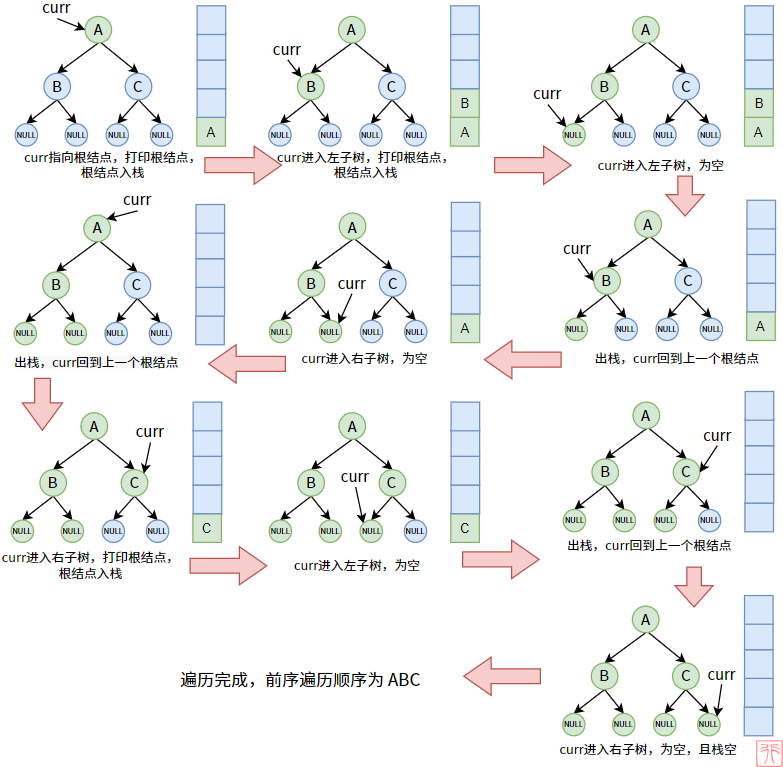【二叉树的概念和原理】中，我们已经看到了，遍历完某个子树时，一定要回到其双亲结点。这种回溯如何实现？可以利用栈的先进后出、后进先出的特点，这个特点能在栈中完美保存结点在树中父子关系，栈顶元素即为当前子树的双亲结点。

/**
* 使用栈实现的先序遍历
*/
void preorder_traversal_by_stack(TreeNode *root)
{
//创造并初始化栈
Stack stack;
init_stack(&stack);

TreeNode *curr = root; //辅助指针curr

while (curr != NULL || !stack_is_empty(&stack)) {
while (curr != NULL) {
printf("%c", curr->data); //打印根结点
push(&stack, curr); //根结点入栈
curr = curr->left_child; //进入左子树
}
if (!stack_is_empty(&stack)) {
pop(&stack, &curr); //出栈，回到上一个根结点
curr = curr->right_child; //进入右子树
}
}
}

【后序遍历】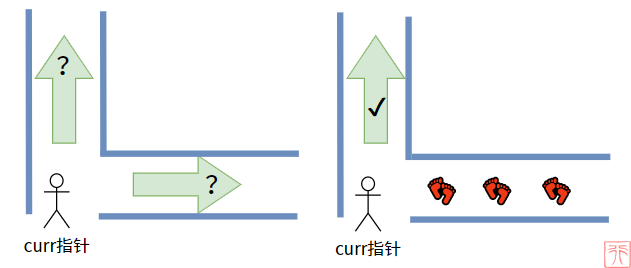curr 指针第二次回到根结点的时候，一看，哦！我的脚印留在那呢！（prev 指针指在右子树那里）curr 指针就直接放心打印根结点了。

/**
* 使用栈实现的后序遍历
*/
void postorder_traversal_by_stack(TreeNode *root)
{
Stack stack;
init_stack(&stack);

TreeNode *curr = root; //辅助指针curr，记录当前访问结点
TreeNode *prev = NULL; //脚印指针prev，记录上一个访问过的结点

while (curr != NULL || !stack_is_empty(&stack)) {
if (curr != NULL) {
push(&stack, curr); //根结点入栈
curr = curr->left_child; //进入左子树
} else {
get_top(&stack, &curr); //读栈顶元素，不是出栈
//右子树不为空，且右子树没被遍历
if (curr->right_child != NULL && curr->right_child != prev) {
curr = curr->right_child; //进入右子树
push(&stack, curr); //根结点入栈
curr = curr->left_child; //进入左子树
} else { //右子树已被遍历或者右子树为空，可以打印根结点了
pop(&stack, &curr); //根结点出栈
printf("%c", curr->data); //打印根结点
prev = curr; //记录
curr = NULL; //置空，进入下一轮循环
}
}
}
}

# 4. 总结